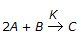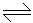# Chemical Engineering - Chemical Reaction Engineering

### Exercise :: Chemical Reaction Engineering - Section 9

31.

The order of the chemical reaction,, whose rate equation is given as -rA = KCA2. CB is

 A. 0 B. 1 C. 2 D. 3

Explanation:

No answer description available for this question. Let us discuss.

32.

What is the dispersion number for a plug flow reactor ?

 A. 0 B. ∞ C. 1 D. -1

Explanation:

No answer description available for this question. Let us discuss.

33.

The decomposition of A into B is represented by the exothermic reaction, A2B. To achieve maximum decomposition, it is desirable to carry out the reaction.

 A. at high P and high T. B. at low P and high T. C. at low P and low T. D. at high P and low T.

Explanation:

No answer description available for this question. Let us discuss.

34.

The catalytic converter for conversion of SO2 to SO3 by contact process should have a feed with SO2 content between

 A. 2-5% B. 7-10% C. 12-15% D. 20-25%

Explanation:

No answer description available for this question. Let us discuss.

35.

If the pore diffusion controls in a catalytic reaction, the apparent activation energy Ea is equal to

 A. the intrinsic activation energy E. B. (E + ED) where ED is activation due to diffusion. C. (E + ED)/2 D. ED/2